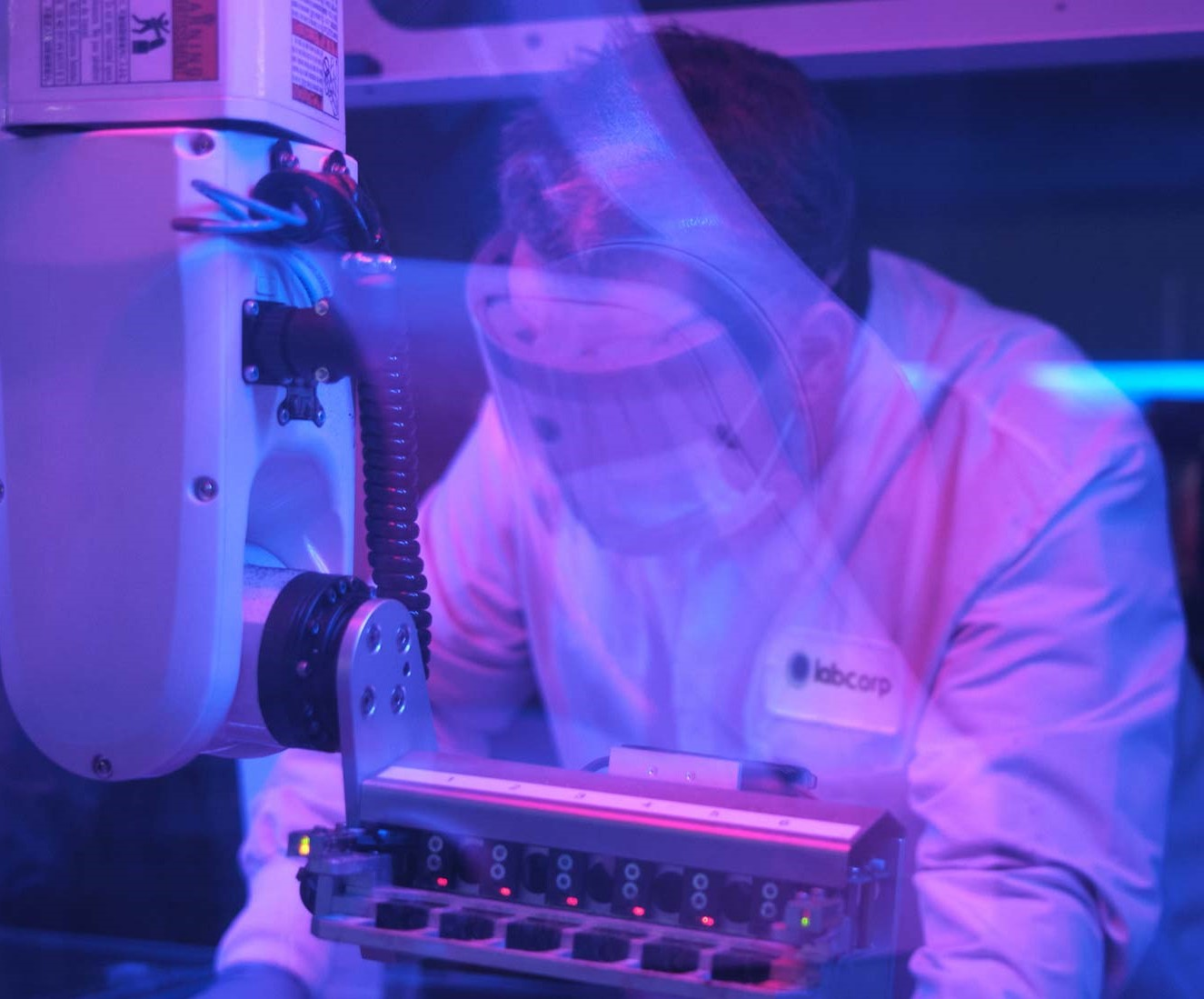Labcorp and its Specialty Testing Group, a fully integrated portfolio of specialty and esoteric testing laboratories.# Cerebrospinal Fluid Amino Acid Reference Intervals

Analyte

0−30 d

(μmol/L)

31 d−11 m

(μmol/L)

1 y−17 y

(μmol/L)

>17 y

(μmol/L)

Alanine (α-alanine)

16.7−82.9

17.2−96.0

15.6−91.1

13.2−132.3

β-Alanine

0.0−1.1

0.0−1.2

0.0−1.2

0.0−2.7

γ-Aminobutyric acid (GABA)

0.0−2.1

0.0−5.7

0.0−6.2

0.0−4.3

Arginine

4.5−50.3

8.4−56.3

8.8−51.9

9.2−54.7

Asparagine

1.4−26.5

3.4−22.8

2.9−21.9

1.7−26.9

Aspartic acid

0.0−17.6

0.0−13.3

0.0−12.7

0.0−14.6

Citrulline

1.0−12.3

1.0−12.0

0.9−10.4

0.7−11.1

Cystine

0.0−4.3

0.0−5.2

0.0−4.8

0.0−13.0

Glutamic acid

1.3−61.3

0.0−58.4

0.0−104.0

0.0−84.0

Glutamine

80.7−1247.3

110.8−788.3

111.5−846.7

131.1−660.1

Glycine

2.7−44.5

2.4−39.7

2.3−34.1

2.3−72.7

Histidine

6.9−61.2

7.3−42.4

7.5−39.0

6.0−38.1

Isoleucine

2.7−26.4

2.9−19.7

2.3−19.1

1.8−26.3

Leucine

6.9−57.3

7.0−37.5

6.6−38.1

5.0−60.7

Lysine

10.7−71.8

11.2−60.7

10.3−57.4

11.5−84.9

Methionine

1.4−20.4

1.3−14.7

1.2−13.5

1.3−14.2

Ornithine

1.7−30.3

2.5−33.0

1.9−30.2

1.9−23.6

Phenylalanine

6.1−55.7

5.5−34.9

5.7−36.0

4.0−46.4

Serine

20.0−130.5

22.3−114.4

17.5−110.3

10.4−57.8

Taurine

3.5−42.9

3.9−34.1

3.1−31.2

2.5−30.2

Threonine

17.2−186.9

14.3−128.9

11.5−120.2

13.2−90.8

Tyrosine

5.8−64.8

5.3−46.6

4.8−42.9

3.6−38.4

Valine

10.5−67.6

10.3−55.3

8.7−53.3

6.8−87.2

## Provider?

Please login to Labcorp Link to pay your bill.